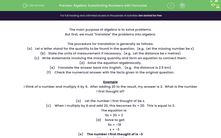# Create and Solve Equations for Word Problems

In this worksheet, students will use algebra to solve word problems.Key stage:  KS 3

Curriculum topic:   Algebra

Curriculum subtopic:   Substitute Numerical Values for Formulae/Expressions

Popular topics:   Algebra worksheets

Difficulty level:#### Worksheet Overview

The main purpose of algebra is to solve problems.

Using equations to solve problems can be useful when we are given a word problem.

Example

I think of a number and multiply it by 6.  After adding 20 to the result, my answer is 2.  What is the number I first thought of?

Let the number I first thought of be x

When I multiply by 6 and add 20, this becomes 6x + 20.  This is equal to 2.

The equation is:  6x + 20 = 2

Solve to get:

6x = -18

x = -3

The number I first thought of is -3

We can check our answer by putting it back into the equation:

-3 × 6 = -18

-18 + 20 = 2 so it works.

Let's have a go at some questions now.

### What is EdPlace?

We're your National Curriculum aligned online education content provider helping each child succeed in English, maths and science from year 1 to GCSE. With an EdPlace account you’ll be able to track and measure progress, helping each child achieve their best. We build confidence and attainment by personalising each child’s learning at a level that suits them.

Get started